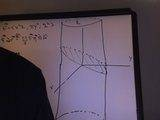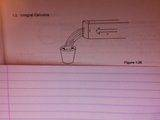# Related Rates Problem

## Homework Statement

#19## Homework Equations

Implicit Differentiation

## The Attempt at a Solution

I have a diagram and I am using the info given to establish some relationships. I guess my main concern is whether I have established a correct relationship between r and y as to eliminate one of them. If I have, am having trouble differentiating the following

V=(pi/3)y^2(3r-y) which after substitution looks like

V=(pi/3)y^2(3sqrt(26y-y^2)-y)

Does this look like I am on the right track to find both dy/dt and dr/dt at y=8...or am I just making things difficult?

Casey

## Answers and Replies

lalbatros
Why don't you calculate V' ?
Obviously V' should be related to y'.
Actually you should have V' = S y', where S is the free surface of water.
Indeed: if the level decreases by dy, the volume decreases by dV=S dy.

But you should not worry to calculate S geometrically, just differentiate V and see what you get.
If you like (geometry), you can also check that your result fits with the free surface.

Also, don't forget to check your result on special cases!
If y=0 you should have V'=0 too, since you are in the bottom of the (spherical) container.
Similarly, for y=2R, you should also get V'=0 since the "ceiling" is very small then.

Note:
====
Expressing y as a function of V to solve this problem is much more complicated.
You would have to solve of polynomial of degree 3!

Last edited:
I am not sure that I follow. I don't think that any special cases apply here do they? I am only trying to calculate dy/dt and dr/dt. I am given that that dV/dt is 6; V=(pi/3)y^2(3r-y); the radius of the sphere is 13, but when the water reaches height y=8, the water's radius=r.

Shouldn't I differentiate the formula for V? Since dV/dt=dy/dt*dV/dy, thus I can solve for dy/dt?

But, to differentiate V with respect to y, don't I have to rewrite r in terms of y? (or vice versa and solve for dr/dt?)

lalbatros
In this expression:

V = pi/3 y²(3R-y)

only y is a function of time, other things are constant.
Calculate dV/dt = dV/dy dy/dt, simplify your expression and go back to my first answer.
If you wish post your result for dV/t, I will check if it is ok, I will first check that it fits the special cases.
From you result, you will be able to solve for dy/dt.

I go to bed now and will come here again tomorrow.

$$If R=13 then, V=\frac{\pi}{3}y^2(39-y)$$
$$\rightarrowV=13{\pi}y^2-\frac{\pi}{3}y^3$$
so $$\frac{dV}{dy}=26{\pi}y-{\pi}y^2$$
at $$y=8, \frac{dV}{dy}=144{\pi}$$

$$\frac{dy}{dt}=\frac{\frac{dV}{dt}}{\frac{dv}{dy}}=-\frac{6}{144/{pi}}=-\frac{1}{24\pi}}$$

which when I add the correct units yields the correct answer. I am not sure what the problem was but I figured it out and made my first attempt at using Latex. (I am prouder of the latter!)

By the way, how do I get a space in between "If" and "R" in If R=... using Latex?

Thanks Labs,
Casey

Last edited:
lalbatros
Normal typewriting in Latex is a mystery for me, I am used to write formulas, nothing else.
Your expression $$\frac{dV}{dy}=26{\pi}y-{\pi}y^2$$ give zero for y=0 and for y=2R=26, which is a good indication that you got the right result.
Why did you put the numeric values right from the begining?
Would it not have been more funny if you had the general expression for any value of R? You could have seen it should be proportional to y(2R-y) of course.
Anyway, you got it apparently!